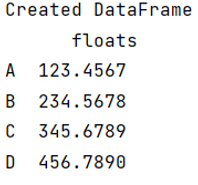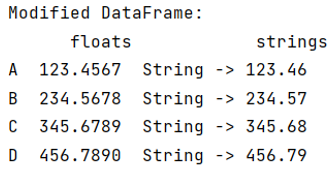# How to display Pandas DataFrame of floats using a format string for columns?

Here, we have to display Pandas DataFrame of floats using a format string for columns.
Submitted by Pranit Sharma, on May 29, 2022

Pandas is a special tool which allows us to perform complex manipulations of data effectively and efficiently. Inside pandas, we mostly deal with a dataset in the form of DataFrame. DataFrames are 2-dimensional data structure in pandas. DataFrames consists of rows, columns and the data.

The most fascinating key point about pandas is that it contains almost every kind of logical and mathematical operations which we can perform on our DataFrame for analysing purpose.

Pandas offers us a feature to convert floats into a format of string. This can be done in multiple ways but here we are going to use map() method.

To work with pandas, we need to import pandas package first, below is the syntax:

```import pandas as pd
```

Let us understand with the help of an example,

```# Importing pandas package
import pandas as pd

# Creating a dictionary
d = {'floats':[123.4567, 234.5678, 345.6789, 456.7890]}

# Creating a DataFrame
df = pd.DataFrame(d,index=['A','B','C','D'])

# Display created DataFrame
print("Created DataFrame\n",df)
```

Output:Here, since all the values of the column floats having the data type float, we are now going to use map() method to convert all the floats into string.

```# Using map method to convert all the floats into string
# Also all the converted values will go into
# another column called strings
df['strings'] = df['floats'].map('String -> {:,.2f} '.format)

# Display modified DataFrame
print("Modified DataFrame:\n",df)
```

Output:What's New (MCQs)

Top Interview Coding Problems/Challenges!

IncludeHelp's Blogs

Languages: » C » C++ » C++ STL » Java » Data Structure » C#.Net » Android » Kotlin » SQL
Web Technologies: » PHP » Python » JavaScript » CSS » Ajax » Node.js » Web programming/HTML
Solved programs: » C » C++ » DS » Java » C#
Aptitude que. & ans.: » C » C++ » Java » DBMS
Interview que. & ans.: » C » Embedded C » Java » SEO » HR
CS Subjects: » CS Basics » O.S. » Networks » DBMS » Embedded Systems » Cloud Computing
» Machine learning » CS Organizations » Linux » DOS
More: » Articles » Puzzles » News/Updates